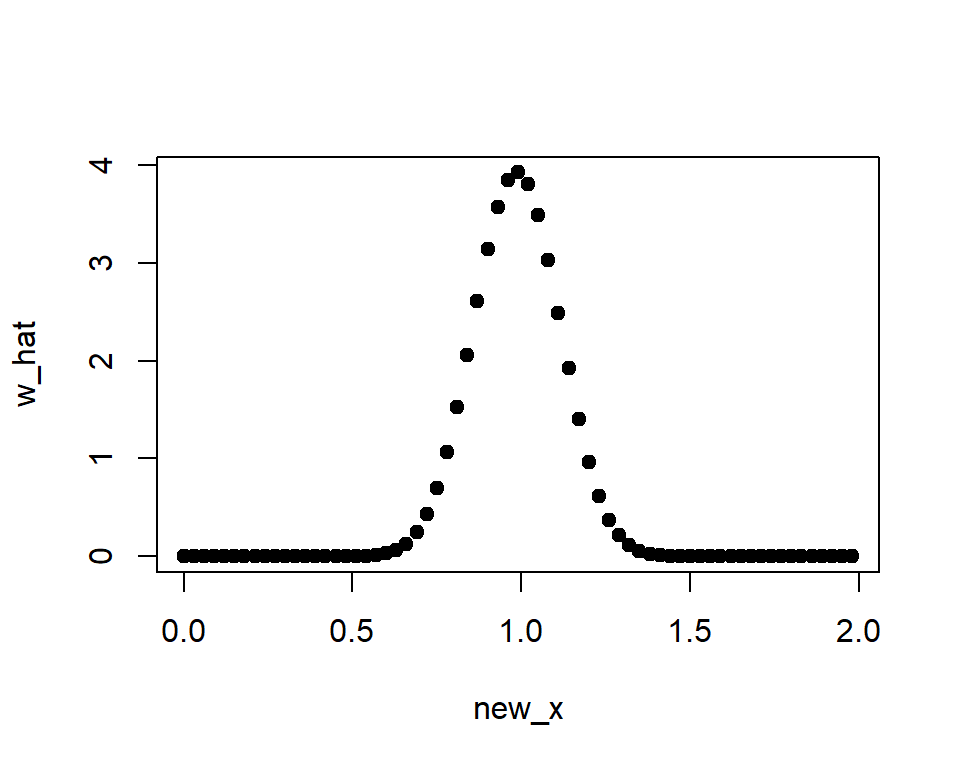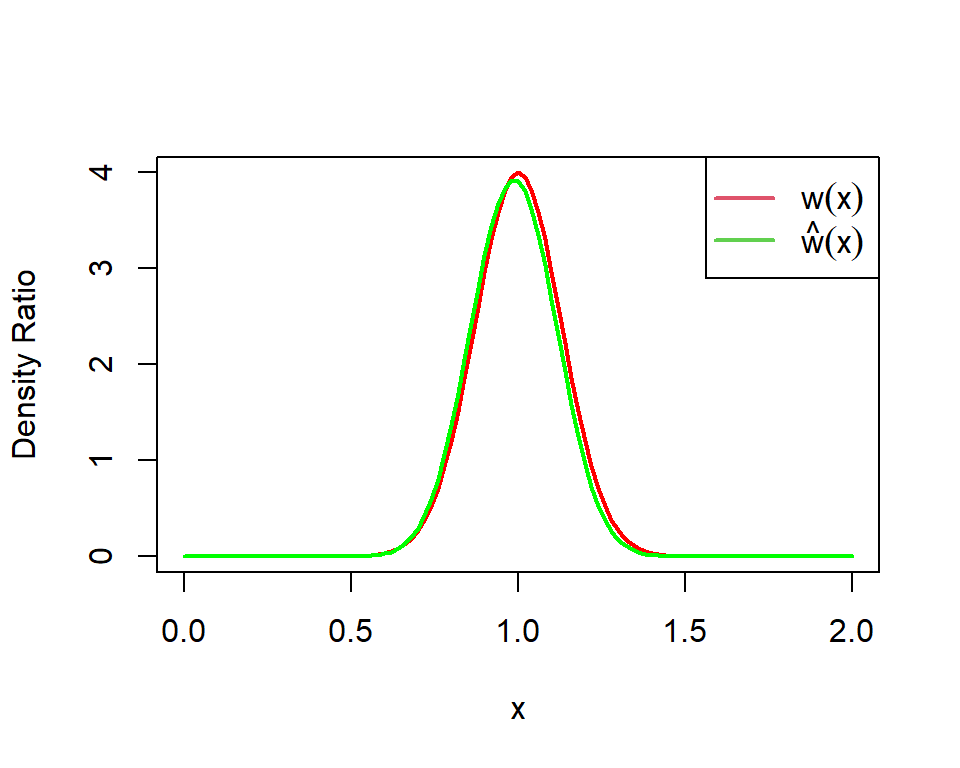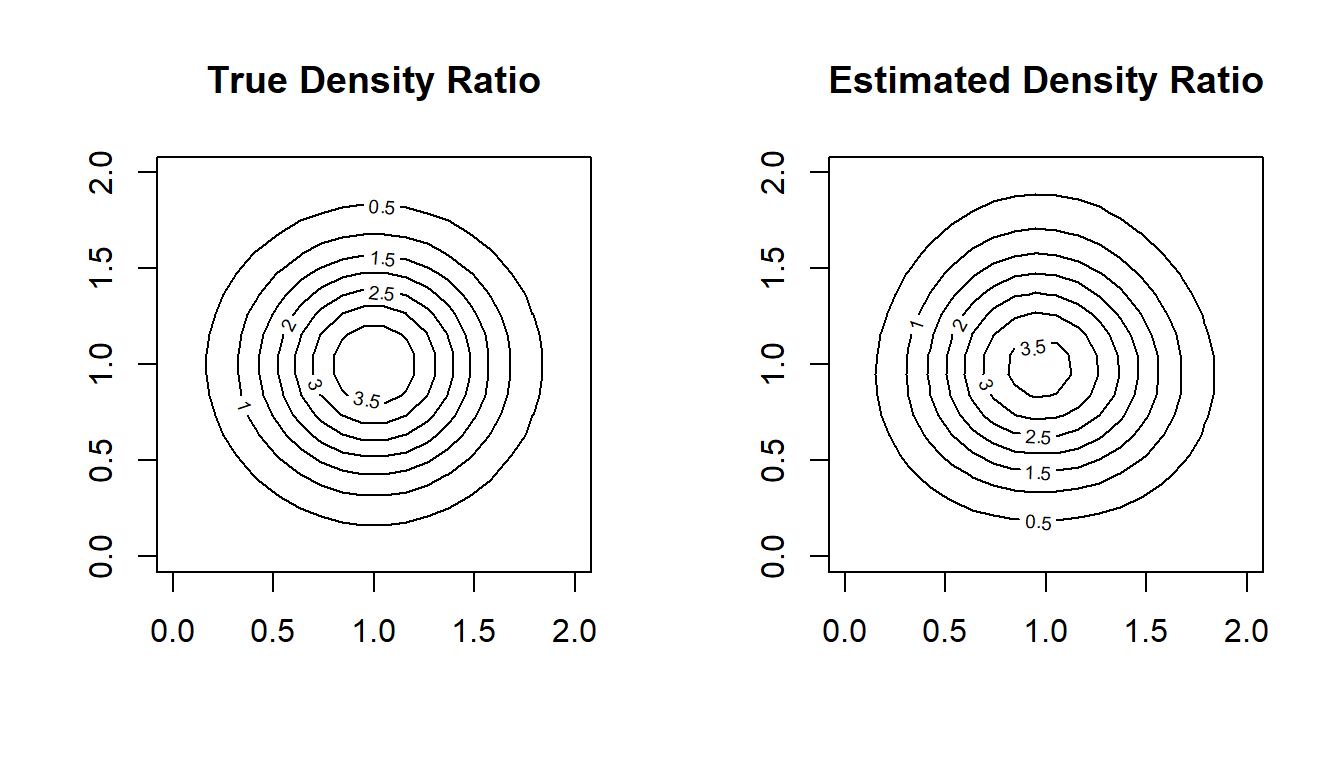# An R Package for Density Ratio Estimation

## 1. Overview

Density ratio estimation is described as follows: for given two data samples `x1` and `x2` from unknown distributions `p(x)` and `q(x)` respectively, estimate `w(x) = p(x) / q(x)`, where `x1` and `x2` are d-dimensional real numbers.

The estimated density ratio function `w(x)` can be used in many applications such as anomaly detection [Hido et al. 2011], change-point detection [Liu et al. 2013], and covariate shift adaptation [Sugiyama et al. 2007]. Other useful applications about density ratio estimation were summarized by [Sugiyama et al. 2012].

The package densratio provides a function `densratio()` that returns an object with a method to estimate density ratio as `compute_density_ratio()`.

For example,

``````set.seed(3)
x1 <- rnorm(200, mean = 1, sd = 1/8)
x2 <- rnorm(200, mean = 1, sd = 1/2)

library(densratio)
densratio_obj <- densratio(x1, x2)``````

The densratio object has a function `compute_density_ratio()` that can compute the estimated density ratio `w_hat(x)` for any d-dimensional input `x` (now d=1).

``````new_x <- seq(0, 2, by = 0.03)
w_hat <- densratio_obj\$compute_density_ratio(new_x)

plot(new_x, w_hat, pch=19)``````In this case, the true density ratio `w(x) = p(x)/q(y) = Norm(1, 1/8) / Norm(1, 1/2)` is known. So we can compare `w(x)` with the estimated density ratio `w-hat(x)`.

``````true_density_ratio <- function(x) dnorm(x, 1, 1/8) / dnorm(x, 1, 1/2)

plot(true_density_ratio, xlim=c(0, 2), lwd=2, col="red", xlab = "x", ylab = "Density Ratio")
plot(densratio_obj\$compute_density_ratio, xlim=c(0, 2), lwd=2, col="green", add=TRUE)
legend("topright", legend=c(expression(w(x)), expression(hat(w)(x))), col=2:3, lty=1, lwd=2, pch=NA)``````## 2. Installation

You can install the densratio package from CRAN.

``install.packages("densratio")``

You can also install the package from GitHub.

``````install.packages("remotes") # if you have not installed "remotes" package
remotes::install_github("hoxo-m/densratio")``````

The source code for densratio package is available on GitHub at

## 3. Details

### 3.1 Basics

The package provides `densratio()`. The function returns an object that has a function to compute estimated density ratio.

For data samples `x1` and `x2`,

``````set.seed(3)
x1 <- rnorm(200, mean = 1, sd = 1/8)
x2 <- rnorm(200, mean = 1, sd = 1/2)

library(densratio)
densratio_obj <- densratio(x1, x2)``````

In this case, `densratio_obj\$compute_density_ratio()` can compute estimated density ratio.

``````new_x <- seq(0, 2, by = 0.03)
w_hat <- densratio_obj\$compute_density_ratio(new_x)

plot(new_x, w_hat, pch=19)``````### 3.2 Methods

`densratio()` has `method` argument that you can pass `"uLSIF"`, `"RuSLIF"`, or `"KLIEP"`.

• uLSIF (unconstrained Least-Squares Importance Fitting) is the default method. This algorithm estimates density ratio by minimizing the squared loss. You can find more information in [Kanamori et al. 2009] and [Hido et al. 2011].
• RuLSIF (Relative unconstrained Least-Squares Importance Fitting). This algorithm estimates relative density ratio by minimizing the squared loss. You can find more information in [Yamada et al. 2011] and [Liu et al. 2013].
• KLIEP (Kullback-Leibler Importance Estimation Procedure). This algorithm estimates density ratio by minimizing Kullback-Leibler divergence. You can find more information in [Sugiyama et al. 2007].

The methods assume that density ratio are represented by linear model:

• `w(x) = theta_1 * K(x, c_1) + theta_2 * K(x, c_2) + ... + theta_b * K(x, c_b)`

where

• `K(x, c) = exp(-||x - c||^2 / 2 * sigma^2)`

is the Gaussian (RBF) kernel.

`densratio()` performs the following:

• Decides kernel parameter `sigma` by cross-validation,
• Optimizes the kernel weights `theta` (in other words, find the optimal coefficients of the linear model), and
• The parameters `sigma` and `theta` are saved into `densratio` object, and are used when to compute density ratio in the call `compute_density_ratio()`.

### 3.3 Result and Arguments

`densratio()` outputs the result like as follows:

``````#>
#> Call:
#> densratio(x = x1, y = x2, method = "uLSIF")
#>
#> Kernel Information:
#>   Kernel type:  Gaussian
#>   Number of kernels:  100
#>   Bandwidth (sigma):  0.1
#>   Centers:  num [1:100, 1] 0.907 1.093 1.18 1.136 1.046 ...
#>
#> Kernel Weights:
#>   num [1:100] 0.067455 0.040045 0.000459 0.016849 0.067084 ...
#>
#> Regularization Parameter (lambda):  1
#>
#> Function to Estimate Density Ratio:
#>   compute_density_ratio()``````
• Kernel type is fixed as Gaussian.
• Number of kernels is the number of kernels in the linear model. You can change by setting `kernel_num` argument. In default, `kernel_num = 100`.
• Bandwidth (sigma) is the Gaussian kernel bandwidth. In default, `sigma = "auto"`, the algorithm automatically select an optimal value by cross validation. If you set `sigma` a number, that will be used. If you set `sigma` a numeric vector, the algorithm select an optimal value in them by cross validation.
• Centers are centers of Gaussian kernels in the linear model. These are selected at random from the data sample `x1` underlying a numerator distribution `p(x)`. You can find the whole values in `result\$kernel_info\$centers`.
• Kernel Weights are `theta` parameters in the linear kernel model. You can find these values in `result\$kernel_weights`.
• Function to Estimate Density Ratio is named `compute_density_ratio()`.

## 4. Multi Dimensional Data Samples

So far, the input data samples `x1` and `x2` were one dimensional. `densratio()` allows to input multidimensional data samples as `matrix`, as long as their dimensions are the same.

For example,

``````library(densratio)
library(mvtnorm)

set.seed(3)
x1 <- rmvnorm(300, mean = c(1, 1), sigma = diag(1/8, 2))
x2 <- rmvnorm(300, mean = c(1, 1), sigma = diag(1/2, 2))

densratio_obj_2d <- densratio(x1, x2)
densratio_obj_2d
#>
#> Call:
#> densratio(x = x1, y = x2, method = "uLSIF")
#>
#> Kernel Information:
#>   Kernel type:  Gaussian
#>   Number of kernels:  100
#>   Bandwidth(sigma):  0.316
#>   Centers:  num [1:100, 1:2] 1.257 0.758 1.122 1.3 1.386 ...
#>
#> Kernel Weights:
#>   num [1:100] 0.0756 0.0986 0.059 0.0797 0.0421 ...
#>
#> Regularization Parameter (lambda):  0.3162278
#>
#> Function to Estimate Density Ratio:
#>   compute_density_ratio()``````

In this case, as well, we can compare the true density ratio with the estimated density ratio.

``````true_density_ratio <- function(x) {
dmvnorm(x, mean = c(1, 1), sigma = diag(1/8, 2)) /
dmvnorm(x, mean = c(1, 1), sigma = diag(1/2, 2))
}

N <- 20
range <- seq(0, 2, length.out = N)
input <- expand.grid(range, range)
w_true <- matrix(true_density_ratio(input), nrow = N)
w_hat <- matrix(densratio_obj_2d\$compute_density_ratio(input), nrow = N)

par(mfrow = c(1, 2))
contour(range, range, w_true, main = "True Density Ratio")
contour(range, range, w_hat, main = "Estimated Density Ratio")``````• A Python Package for Density Ratio Estimation
• APPEstimation: Adjusted Prediction Model Performance Estimation
• Hido, S., Y. Tsuboi, H. Kashima, M. Sugiyama, and T. Kanamori. Statistical outlier detection using direct density ratio estimation. Knowledge and Information Systems, 2011.
• Kanamori, T., S. Hido, and M. Sugiyama. A least-squares approach to direct importance estimation. Journal of Machine Learning Research, 2009.
• Liu, S., M. Yamada, N. Collier, M. Sugiyama. Change-point detection in time-series data by relative density-ratio estimation. Neural Net, 2013
• Sugiyama, M., S. Nakajima, H. Kashima, P. von Bünau, and M. Kawanabe. Direct importance estimation with model selection and its application to covariate shift adaptation. NIPS 2007.
• Sugiyama, M., T. Suzuki, and T. Kanamori. Density ratio estimation in machine learning. Cambridge University Press, 2012.
• Yamada, M., T. Suzuki, T. Kanamori, H. Hachiya, and M. Sugiyama. Relative density-ratio estimation for robust distribution comparison. NIPS 2011.